Courses

# Test: Hybridisation

## 22 Questions MCQ Test Chemistry Class 11 | Test: Hybridisation

Description
This mock test of Test: Hybridisation for JEE helps you for every JEE entrance exam. This contains 22 Multiple Choice Questions for JEE Test: Hybridisation (mcq) to study with solutions a complete question bank. The solved questions answers in this Test: Hybridisation quiz give you a good mix of easy questions and tough questions. JEE students definitely take this Test: Hybridisation exercise for a better result in the exam. You can find other Test: Hybridisation extra questions, long questions & short questions for JEE on EduRev as well by searching above.
QUESTION: 1

### Direction (Q. Nos. 1-11) This section contains 11 multiple choice questions. Each question has four choices (a), (b), (c) and (d), out of which ONLY ONE option is correct. Q.  Assuming that Hund's rule is violated, the bond order and magnetic nature of the diatomic molecule B2 is

Solution:

Molecular orbital configuration of B2 as per the condition of violation of hund's rule will be .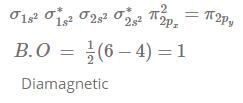QUESTION: 2

### Which of the following statement is incorrect regarding NO2 molecule?

Solution:

NO2 molecule has unpaired electrons which are responsible for its brown colour and paramagnetic behavior.

QUESTION: 3

### Molecule MX3 (atomic number M < 21) has zero dipole moment, the sigma bonding orbitals used by M are

Solution:

Molecule has zero dipole moment, thus it does not have lone pair. Hence, non-polar.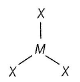Thus, (M — X) bonds indicate sp2-hybridisation.

QUESTION: 4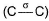bond in between (C2— C3) vinyl acetylene is formed by ...... overlapping

Solution:

Vinyl acetylene is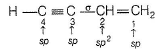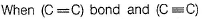bond are at equal position, numbering of C-chains based on IUPAC nomenclature is done from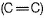side.

QUESTION: 5

Hydrogen bonds are formed in many compounds e.g., H2O, HF, NH3 . The boiling point of such compounds depends to a large extent on the strength of hydrogen bond and the number of hydrogen bonds. The correct decreasing order of the boiling points of above compounds is :

Solution:

H2​O>HF>NH3​

Strength of hydrogen bonding depends on the size and electronegativity of the atom.

Smaller the size of the atom, greater is the electronegativity and hence stronger is the H−bonding. Thus, the order of strength of H-bonding is H...F>H...O>H...N.

But each HF molecule is linked only to two other HF molecules while each H2O molecule is linked to four other H2​O molecules through H−bonding.

Hence, the decreasing order of boiling points is H2​O>HF>NH3​.

QUESTION: 6

Considering the state of hybridisation of C-atoms,select the molecule among the following which is linear

Solution:

Molecules with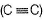have bond angle 180°. In (d) there is also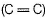and is not symmetrical.
(c) is symmetrical w.r.t.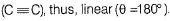QUESTION: 7

In which one of the following species the central atom has the type of hybridisation which si not the same as that present in the other three?

Solution:

Molecules having the same number of hybrid orbitals, have same hybridisation and number of hybrid oebitals.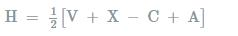where,

V= number of valance electrons of central atom

X = number of monovalent atoms

C= charge on cation

A = charge on anion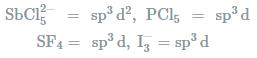QUESTION: 8

BF3 is electron deficient (Lewis acid) and NH3 is electron-rich (Lewis acid). In 1 : 1 complex of BF3 and NH3, then coordination geometry and hybridisation of N and B atoms are :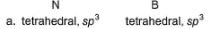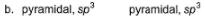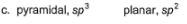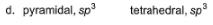Solution: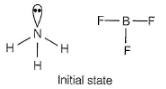After 1 :1 complex is formed in which NH3 is electron donor and BF3 is electron acceptor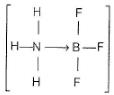Each atom (N, B) both are sp3 hybridised. Since, lone pairs of N-atoms are also involved in bonding, hence,both are sp3 hybridized and tetrahedral.

QUESTION: 9

If there are five electron pairs in outer shell, then structure and bond angle as predicted by Sidgwick-Powell theory is

Solution:

Three electron pairs are inclined at 120°.
Two electron-pairs are at 90° with the first three.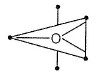Structure is trigonal bipyramidal.

QUESTION: 10

Hybridisation of Acetylene is

Solution:

Since acetylene is made up of triple bond. So the hybridization of carbon in acetylene is sp.

QUESTION: 11

Based on VSEPR model, AX4E representation is for

Solution: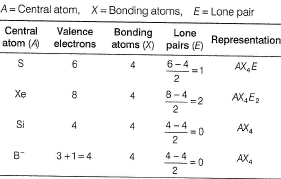QUESTION: 12

Direction (Q. Nos. 12 and 14) This sectionis based on statement I and Statement II. Select the correct answer from the code given below.

Q.

Statement I : BF3 molecule is planar but NF3 is pyramidal.

Statement II : N atom is smaller than B.

Solution: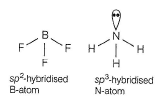(Ip-bp) repulsion make it pyramidal.
Thus, Statement I and II both are correct but Statement II is not the correct explanation of Statement I.

QUESTION: 13

Statement I : B atom is sp2-hybridised in B2H6.
Statement II : There is no lone pair or unpaired electron in B2H6.

Solution:

(d) B2H6 has following types of bonding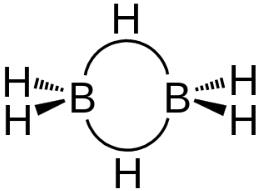Bridging H-atoms and B-atoms are electron deficient. Each B-atom is however, joined to four H-atoms thus sp3-hybridised.
There is no lone pair or unpaired electron.
Thus, Statement I is incorrect but Statement II is correct.

QUESTION: 14

Statement I : BCI3 exists as monomer while AICI3 exists as dimer.

Statement II : Boron atom is too small to form the stable 4-membered ring system

Solution: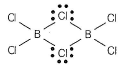Size of B is too small to form stable 4-membered ring system. (Also delocalised n-bonding is disturbed). Hence, dimerofBCI3is not formed.
Dimer AI2CI6 is stable due to stable ring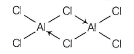Thus, Statements I and II both are correct and Statement II is the correct explanation of Statement

*Multiple options can be correct
QUESTION: 15

Direction (Q. Nos. 15-17) This section contains 3 multiple choice questions. Each question has four choices (a), (b), (c) and (d), out of which ONE or  MORE THANT ONE  is correct.

Q.
Select the correct order of bond lengths of the specified bonds.

Solution: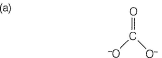Due to resonance (C— O) bond length is larger than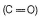but smaller than (C— O).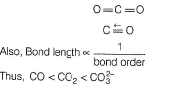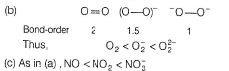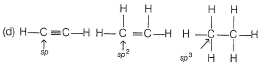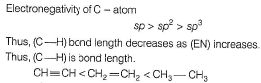*Multiple options can be correct
QUESTION: 16

NH4NO3 and NH4NO2 differ is

Solution: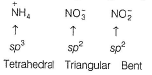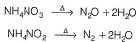(a) Both N in anion are sp2-hybridised.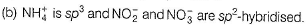(c) Decomposition products are different.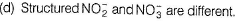*Multiple options can be correct
QUESTION: 17

Select the correct statement(s) about ethene.

Solution: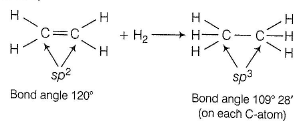(C— H ) bond length < (C— H ) bond length
Nodal, plane of π-bond is located in molecular plane.

QUESTION: 18

Direction (Q. Nos. 18-19) This section contains  a paragraph, each describing theory, experiments, data etc. three Questions related to paragraph have been given.Each question have only one correct answer among the four given options (a),(b),(c),(d).

In certain polar solvents, PCI5 undergoes an ionisation reaction as shown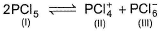Q. Select the incorrect statement (s).

Solution: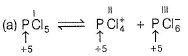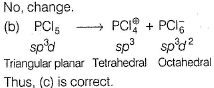QUESTION: 19

In certain polar solvents, PCI5 undergoes an ionisation reaction as shownQ. Number of lone pairs on P in I, il and III are

Solution:

Lone pair on each phosphorus atom = 0

QUESTION: 20

Direction (Q. Nos. 20-21) This section contains 4 multiple choice questions. Each question has four choices (a), (b), (c) and (d), out of which ONE or  MORE THANT ONE  is correct.

Q. Match the following compounds given in Column I with their geometry given is Column II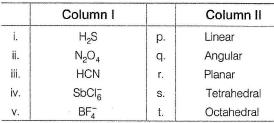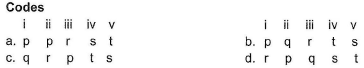Solution: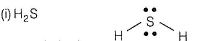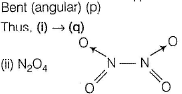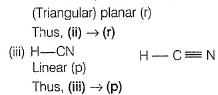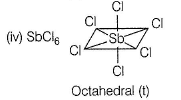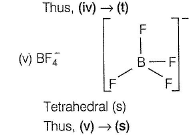QUESTION: 21

Match the processes in Column I with type of changes in hybridisation in Column II.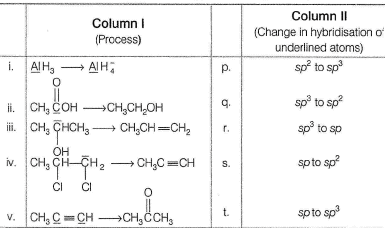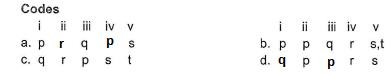Solution: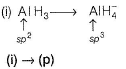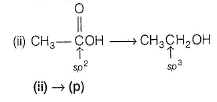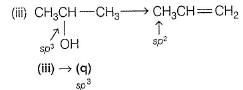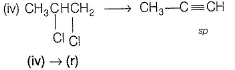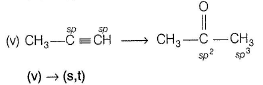*Answer can only contain numeric values
QUESTION: 22

Direction (Q. Nos. 22) This section contains 1 questions. when worked out will result in an integer from 0 to 9 (both inclusive)

Q. Most acidic H in the following compound is attached to C-atoms denoted by at S-N. ( ) ...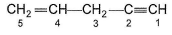Solution:

Electronegativity of
sp3 < sp2 < sp-hybridised C-atom
(Greater the s-character, greater the electronegativity).
(C— H) electron pair is taken out by most (EN) atom most easily, making it is acidic.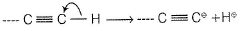• Test